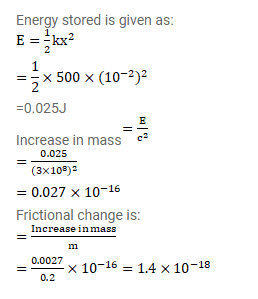# By what fraction does the mass of a spring change

Question:

By what fraction does the mass of a spring change when it is compressed by $1 \mathrm{~cm}$ ? The mass of the spring is $200 \mathrm{~g}$ at its natural length and the spring constant is $500 \mathrm{~N} / \mathrm{m}$.

Solution: